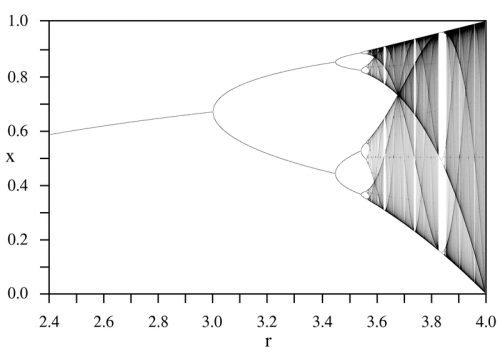## Archive for February, 2009

### Chaos and the Logistic Map

Monday, February 16th, 2009

Linearity is the exception rather than the norm.  We live in a very nonlinear world – for example, it is now known that a sinusoidally driven resistor/diode/inductor combination can exhibit chaotic behavior!  Please refer to this excellent SPS Report.

One of the simplest equations that demonstrates this nonlinear behavior – this chaos – is the “Discrete Logistic Equation” or the “Logistic Map“.  Mathematically, it is given by:

$$x_{k+1}=rx_{k}\left(1-x_{k}\right)$$

This is typically used as a simple model of population growth with finite resources (i.e. food).  $$x_{k+1}$$ represents the population at the next time step, based on that at the previous time step $$x_{k}$$ where $$0\leq x_{k}\leq 1$$ with “growth rate” $$r$$ where $$0\leq r\leq 4$$.  A plot of the logistic map with respect to growth rate is given below for the interval $$2.4\leq r\leq 4.0$$:Using MATLAB, more sophisticated visualization is possible that may give greater insight into what is happening to the population dynamics as the growth parameter is varied.  For example, how the periodic regions return to chaos via a period-doubling bifurcation.  Shown below is the interval $$3.5\leq r\leq 4.0$$:One lesson to take from all this with respect to audio electronics is that simplicity is best!  If a resistor/diode/inductor can result in chaos, then what can the circuitry in an overly complicated amplifier do?  Not only in electronics is simplicity the best, for example in Strunk and White’s The Elements of Style there is the simple mantra: “Omit needless words!”  Vastly different disciplines – same guiding principle.

### Amplifier Power Ratings

Sunday, February 1st, 2009There is much confusion over amplifier power ratings.  There are two primary things to be aware of: what type of measurement a given rating represents and what this means as far as one’s listening experience.  The former is fairly objective while the later is very subjective.

Continuous RMS

This is the most rigorous of the power measurements, although even in this category there are a few “flavors”.  For example, what is “continuous”?  Ten seconds or ten years?  Also, is this at one frequency (e.g. 1kHz), the full audio bandwidth (e.g. 20Hz-20kHz), or the full bandwidth of the amplifier (e.g. 5Hz-100kHz)?

The next question is how appropriate this measurement is for an audio amplifier.  It is probably very appropriate for a precision programmable ac power supply, but I’m not sure how many people listen to continuous full-amplitude sine waves.  Some may feel better knowing their amplifier will be well within its limits with audio material, but as far as actual audio quality, there isn’t much benefit.

Burst Power

Like with continuous RMS there are a few ways of interpreting this measurement.  Is this the peak power of a continuous sine wave, in which case it is double the continuous RMS value, or does this represent the maximum instantaneous power, in which case it is a function of the power supply rail voltage at idle and the lowest allowable load impedance?

There is some merit to this sort of measurement, but the burst interval should be of a reasonable length.  A 20mS burst test is common with very high power amplifiers, such as those from Powersoft or Lab.Gruppen.  Measurement of actual program material indicates that a 200mS interval may be more appropriate – please refer to this AES paper.  Given a reasonable burst interval, this test is probably the most useful for amplifiers intended to reproduce audio material.  Please note that this burst power measurement may be given as either “peak” or “RMS”, with the former having twice the value of the latter.

EIAJ

This is a measurement of the saturated square wave power of the amplifier and is common with consumer and car audio products.  It tends to give an inflated power rating, which is no doubt why it is popular with low-quality audio products.

This test may be useful for giving a feel of what an amplifier is capable of when severely overdriven, but is not really appropriate for high-quality audio products.